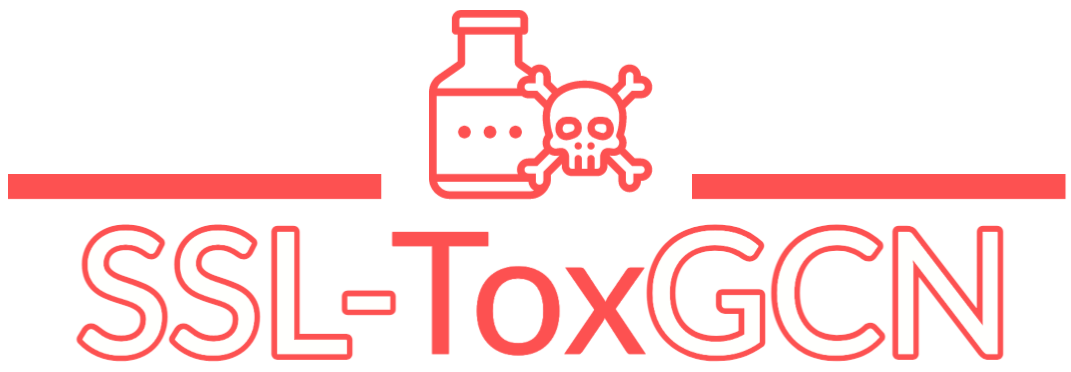## SSL-ToxGCN

A Chemical Toxicity Prediction Model Based on Semi-supervised Learning and Graph Convolutional Neural Network.

## Overview

Currently, the limited availability of annotated toxicity data is the major hurdle for further improving machine learning model performance. In this study, inspired by the success of semi-supervised learning (SSL) algorithms, we propose a Graph Convolution Neural Network (GCN) to predict chemical toxicity and trained the network by the Mean Teacher (MT) SSL algorithm.

Figure 1. The overview of our SSL-ToxGCN model.

As shown in the overview figure (Figure 1), each compound is initially described by a SMILES string. We chose molecule-graph conversion tool from Deep Graph Library (DGL) to convert molecules from SMILES to graphs. For each molecule, the connectivity of atoms is stored in the adjacency matrix (N*N) and the physicochemical properties of each atom (node features) are encoded into a feature matrix (N*74) in binary or numerical form. The detail of atom physicochemical properties can be found in Table 1.

Table 1. Atom features provided by the molecule-graph conversion tool from Deep Graph Library.

The framework of our GCN model consists two parts, encoder and classifier. The encoder extracts and updates node representations through several graph convolutional layers (Graph Conv). In addition, there is a dropout layer after each Graph Conv layer to provide additional noise to the molecular representations. The last layer of the encoder merges all nodes features into a tensor by using max-pooling and weighted sum operations. For the classifier, we used the classifier provided in DGL which contains two layers perceptron (MLP) with a dropout layer and a batch normalization layer.

The prediction result consists of 12 endpoints, including 7 nuclear receptor signals (NR-AR, NR-AhR, NR-AR-LBD, NR-ER, NR-ER-LBD, NR-Aromatase, NR-PPAR-gamma) and 5 stress response indicators (SR-ARE, SRATAD5, SR-HSE, SR-MMP, SR-p53). The detail of these endpoints could be found at: https://tripod.nih.gov/tox21/challenge/about.jsp. Our model will give predictions in binary format, i.e., “1” represents positive response for a particular endpoint and “0” represents negative response.

## Model Performance

We adopted the scaffold splitting method with splitting ratios of 0.8:0.1:0.1 to divide the dataset into training, validation and test sets. All experiments were repeated 5 times on test set to observe the variability of the results and obtain an accurate measure of model performance through the average ROC-AUC score.

Figure 2. Comparison of AUC scores between SL-GCN, SSL-GCN and CM models Comparison of the best models from conventional methods (CM), supervised GCN (SL-GCN), and the semi-supervised GCN (SSL-GCN) on twelve toxicity prediction tasks. The mean and standard deviation are obtained from the 5-repeat experiments.

Figure 2 compares the best CM, SL-GCN and SSL-GCN models. As can be clearly seen, SSL-GCN can produce models with greater predictive potential than CM and SL-GCN in all toxicity prediction tasks. SSL-GCN models consistently outperform their SL-GCN and CM counterparts, the ROC-AUC scores improving between 1% and 13%.

Overall, the optimal SSL-GCN models achieve average ROC-AUC score of 0.7571 with standard deviation 0.0084 on 12 prediction tasks. Here, we embed these optimal SSL-GCN models into this web server for the convenience of all users.